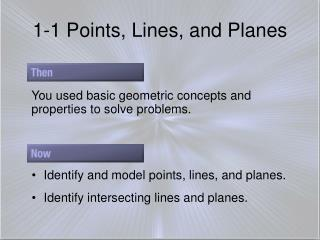DownloadDownload Presentation1-1 Points, Lines, and Planes

# 1-1 Points, Lines, and Planes

Download Presentation## 1-1 Points, Lines, and Planes

- - - - - - - - - - - - - - - - - - - - - - - - - - - E N D - - - - - - - - - - - - - - - - - - - - - - - - - - -
##### Presentation Transcript

1. 1-1 Points, Lines, and Planes You used basic geometric concepts and properties to solve problems. • Identify and model points, lines, and planes. • Identify intersecting lines and planes.

2. point • line • plane • collinear • coplanar • Intersection • space What do you think are basic geometry figures?

3. Point Definition • A point is a location. It has neither shape nor size. • It is named using 1 capital letter. (read point A) A

4. Line Definition • A line is made up of points and has no thickness or width. • There is exactly one line through any two points. • Lines are names with 2 letters representing points on the line or one lower case script letter. written A B

5. Plane Definition • A plane is a flat surface made up of points that extends infinitely in all directions. • There is exactly one plane through any 3 points not on the same line. • It is named by 1 capital script letter or 3 points not all on the same line. A C B K

6. More Definitions • Collinear points are points that lie on the same line. (look for the word “line”) • Noncollinear points do not lie on the same line. • Coplanar points are points that lie in the same plane. (look for the word “plane”) • Noncoplanar points do not lie in the same plane

7. p. 5

8. Answer: The line can be named as line a. There are three points on the line. Any two of the points can be used to name the line. Name Lines and Planes A. Use the figure to name a line containing point K.

9. Answer: The plane can be named as plane B. You can also use the letters of any three noncollinearpoints to name the plane.plane JKM plane KLM plane JLM Name Lines and Planes B. Use the figure to name a plane containing point L. The letters of each of these names can be reordered to create other acceptable names for this plane. For example, JKM can also be written as JMK, MKJ, KJM, KMJ, and MJK. There are 15 different three-letter names for this plane.

10. A.line X B.line c C.line Z D. A. Use the figure to name a line containing the point X.

11. A. Name the geometric shape modeled by a 10  12 patio. Answer: The patio models a plane. B. Name the geometric shape modeled by a button on a table. Answer: The button on the table models a point on a plane. A. Name the geometric shape modeled by a colored dot on a map used to mark the location of a city. A. Point B. line segment C. plane D. none of the above

12. Draw a surface to represent plane R and label it.

13. Draw a line anywhere on the plane.

14. Draw dots on the line for point A and B. Label the points.

15. Label the intersection point of the two lines as P.

17. A. B.C.D. A. Choose the best diagram for the given relationship. Plane D contains line a, line m, and line t, with all three lines intersecting at point Z. Also, point F is on plane D and is not collinear with any of the three given lines.

18. Answer: There are an infinite number of points that are collinear with Q and R. In the graph, one such point is T(1, 0).

19. A. B. C.D.

20. Last Definitions • Intersection - the set of points common to 2 or more geometric figures. • Undefined terms - terms that are only explained by using examples and descriptions. • Defined terms – terms that are explained by using undefined terms and/or other defined terms. • Space – a boundless, 3-dimensional set of all points.

21. A. How many planes appear in this figure? Answer: There are two planes: plane S and plane ABC.

22. B. Name three points that are collinear. Answer: Points A, B, and D are collinear.

23. C. Are points A, B, C, and D coplanar? Explain. Answer: Points A, B, C, and D all lie in plane ABC, so they are coplanar.

24. Answer: The two lines intersect at point A.

25. A. How many planes appear in this figure? A. one B. two C. three D. four

26. B. Name three points that are collinear. A.B, O, and X B.X, O, and N C.R, O, and B D.A, X, and Z

27. A. point X B. point N C. point R D. point A

28. Assignment 1-1 p. 8. 13-18, 20, 32-38 even